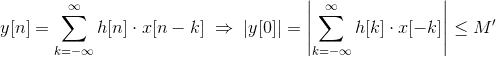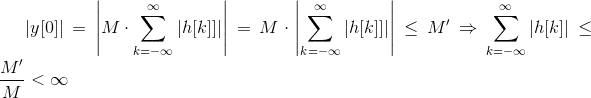# BIBO Stable if and only if impulse response is summable

• Bipolarity
In summary, if a linear time invariant system is BIBO stable, then its impulse response is summable. This is easily proven if the system is FIR, and does not depend on the continuity of the input signal.

#### Bipolarity

It is theorem of any course on signals that a linear time invariant system, whether in discrete or continuous time, is BIBO stable if and only if its impulse response is summable. The fact that summability implies BIBO stability is easy to prove. In fact, it's on the wikipedia page of BIBO stability: http://en.wikipedia.org/wiki/BIBO_stability

I've been trying to prove the converse, that is, if a system is BIBO stable, it is summable. I was able to prove it if the system is FIR:
Let H be an FIR LTI system that is BIBO stable. Then for any input signal x(t) bounded by M, i.e. |(x(t)| < M for all t, the response y(t) is bounded, i.e. |y(t)|<M'.

If the input is the impulse (which is clearly bounded), then output is the impulse response, h(t), which must also be bounded since we assumed BIBO stable.

So |h(t)| < M' for some M'. Since the system is FIR, h(t) has finite duration, so ## \int^{\infty}_{-\infty} |h(t)| dt ## exists. Hence the system is summable.

But is this true even if the system isn't FIR? If so, how might I prove this?

Thanks!

BiP

Bipolarity said:
It is theorem of any course on signals that a linear time invariant system, whether in discrete or continuous time, is BIBO stable if and only if its impulse response is summable. The fact that summability implies BIBO stability is easy to prove. In fact, it's on the wikipedia page of BIBO stability: http://en.wikipedia.org/wiki/BIBO_stability

I've been trying to prove the converse, that is, if a system is BIBO stable, it is summable. I was able to prove it if the system is FIR:
Let H be an FIR LTI system that is BIBO stable. Then for any input signal x(t) bounded by M, i.e. |(x(t)| < M for all t, the response y(t) is bounded, i.e. |y(t)|<M'.
The converse is also true.
Suppose a BIBO-stable LTI system. For a discrete-time case this means:

(a) The system responses with output y[n] to the input x[n]
(b) 0<|x[n]|≤M< , 0<|y[n]|≤M'<∞ for every n

From definition and discrete-time signals theory we have:Without loss of generality (since |x[n]|) we can put x[-k]=-sign(h[k]) and obtain:IOW, h[k] is absolutely summable which concludes the proof.
Showing same thing for continuous systems I leave for mathematical exercise.

zoki85 said:
... we can put x[-k]=-sign(h[k]) and obtain:
Noticed typo here. Of course, it should be x[-k] = M⋅sign(h[k]).
Anyway, the excersise of proving the converse is of no importance to engineers. We are primarily interested in the question if system is stable or not .